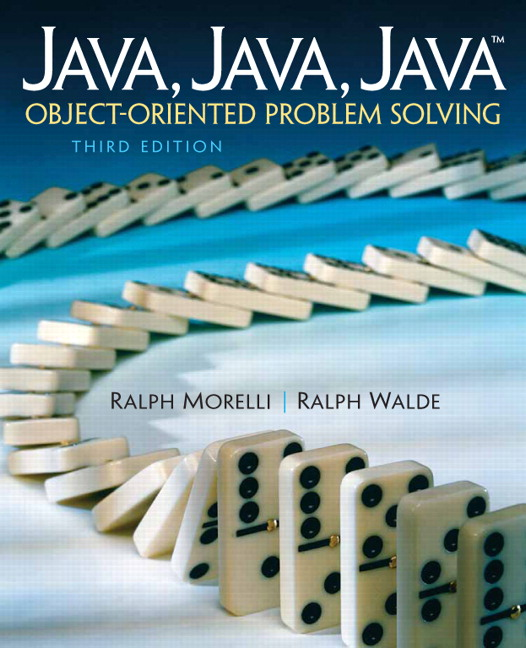# Java, Java, Java: Object-Oriented Problem Solving, 2022E

## Section5.10Example: Character Conversions

Another interesting implication of representing the characters as integers is that we can represent various character operations in terms of integer operations. For example, suppose we want to capitalize a lowercase letter. Figure 5.9.1. shows that the entire sequence of lowercase letters ('a''z') is displaced by 32 from the sequence of uppercase letters ('A''Z'). So we can convert any lowercase letter into its corresponding uppercase letter by subtracting 32 from its integer value, provided we perform an explicit cast on the result. When we perform the cast (char) ('a' - 32 ), the resulting value is 'A', as the following example shows:
(char)('a' - 32)              ==>  'A'

In evaluating 'a' - 32Java will promote ‘a’ to an int and then perform the subtraction. Thus, a step-by-step evaluation of the expression would go as follows:
Step 1. (char)((int)'a' - 32)// Promote 'a' to int
Step 2. (char)(97 - 32)      // Subtract
Step 3. (char) (65)          // Cast result to a char
Step 4. 'A'                  // Results in 'A'

Similarly, we can convert an uppercase letter into the corresponding lowercase letter by simply adding 32 to its integer code and casting the result back to a char:
(char)('J' + 32)              ==>  'j'

We can group these ideas into a method that performs conversion from lowercase to uppercase:
char toUpperCase(char ch)
{
if ((ch >= 'a') && (ch <= 'z'))
return ch - 32 ;  // Error: can't return an int
return ch;
}

This method takes a single char parameter and returns a char value. It begins by checking if ch is a lowercase letter—that is, if ch falls between ‘a’ and ‘z’ inclusive. If so, it returns the result of subtracting 32 from ch. If not, it returns ch unchanged.
However, the method contains a syntax error that becomes apparent if we trace through its steps. If we invoke it with the expression toUpperCase('b'), then since ‘b’ is between ‘a’ and ‘z’, the method will return ‘b’ $$-$$ 32. Because the integer value of ‘b’ is 98, it will return 98 $$-$$ 32 or 66, which is the integer code for the character ‘B’. However, the method is supposed to return a char, so this last statement will generate the following syntax error:
Incompatible type for return. An explicit cast needed
to convert int to char.
>>    return ch - 32 ;
>>    ^

In order to avoid this error, the result must be converted back to char before it can be returned:
char toUpperCase (char ch)
{
if ((ch >= 'a') && (ch <= 'z'))
return (char)(ch - 32);  // Explicit cast
return ch;
}

Another common type of conversion is to convert a digit to its corresponding integer value. For example, we convert the character ‘9’ to the integer 9 by making use of the fact that the digit ‘9’ is 9 characters beyond the digit ‘0’ in the lexical order. Therefore, subtracting ‘0’ from ‘9’ gives integer 9 as a result:
('9' - '0')  ==> (57 - 48) ==>   9

More generally, the expression $$ch -$$ ‘0’ will convert any digit, ch, to its integer value. We can encapsulate these ideas into a method that converts any digit into its corresponding integer value:
int digitToInteger(char ch)
{
if ((ch >= '0') && (ch <= '9'))
return ch - '0';
return -1 ;
}

This method takes a single char parameter and returns an int. It first checks that ch is a valid digit, and if so, it subtracts the character ‘0’ from it. If not, the method just returns $$-1\text{,}$$ which indicates that the method received an invalid input parameter. Obviously, when an object invokes this method, it should first make sure that the value it passes is in fact a digit.
The program shown in Listing 5.10.1 illustrates several of the ideas discussed in this section. Note that both the digitToInteger() and toUpperCase() are declared static. This allows us to call them directly from the (static) main() method, a useful and justifiable shortcut if, as in this case, we are just testing the methods.